CBSE Class 12 Sample Paper for 2023 Boards

Class 12
Solutions of Sample Papers and Past Year Papers - for Class 12 Boards

## 𝑐𝑜𝑠𝜃 = |a 1 a 2 + b 1 b 2 + c 1 c 2 |/(√a 1 2 + b 1 2 + c 1 2 √a 2 2 + b2 2 . + c 2 2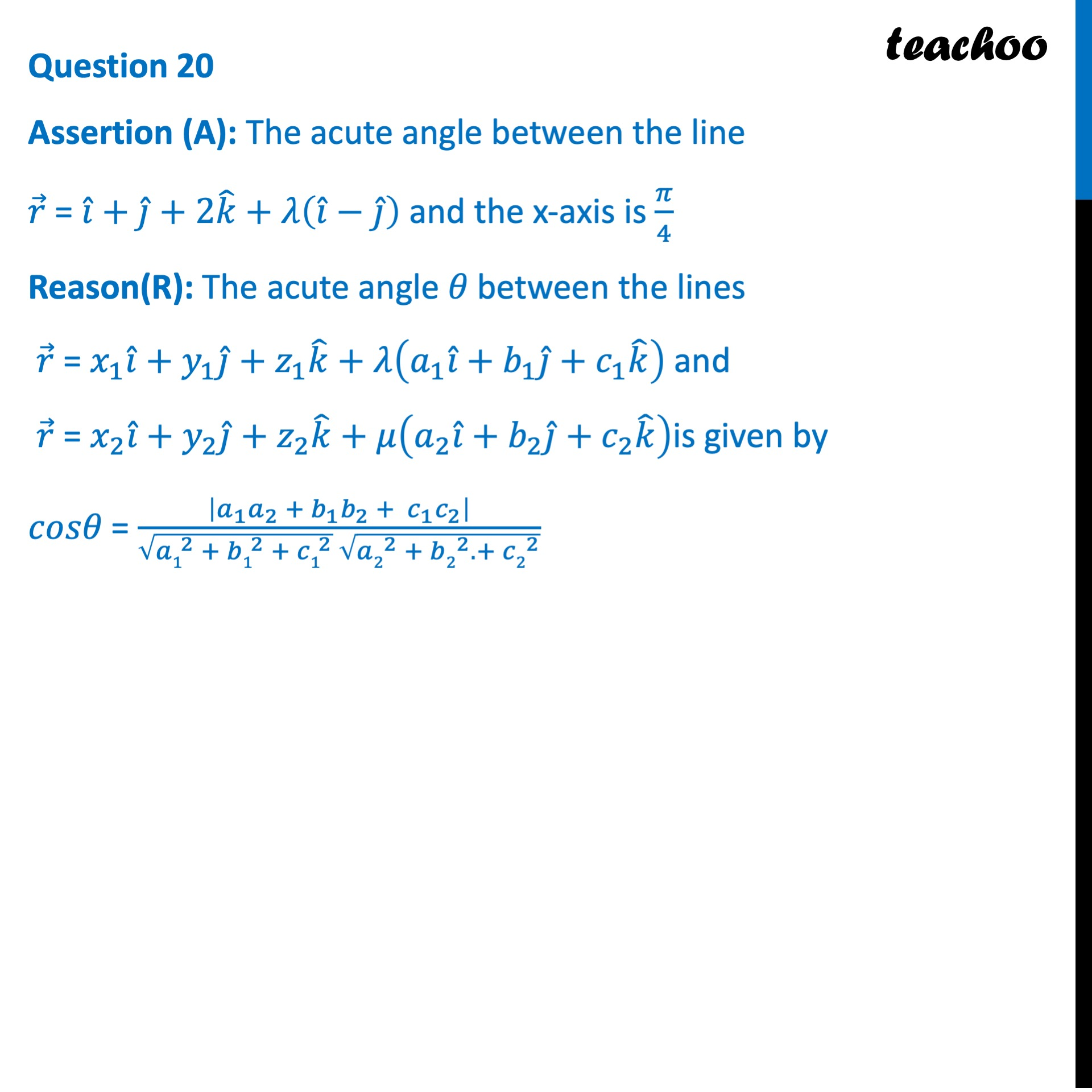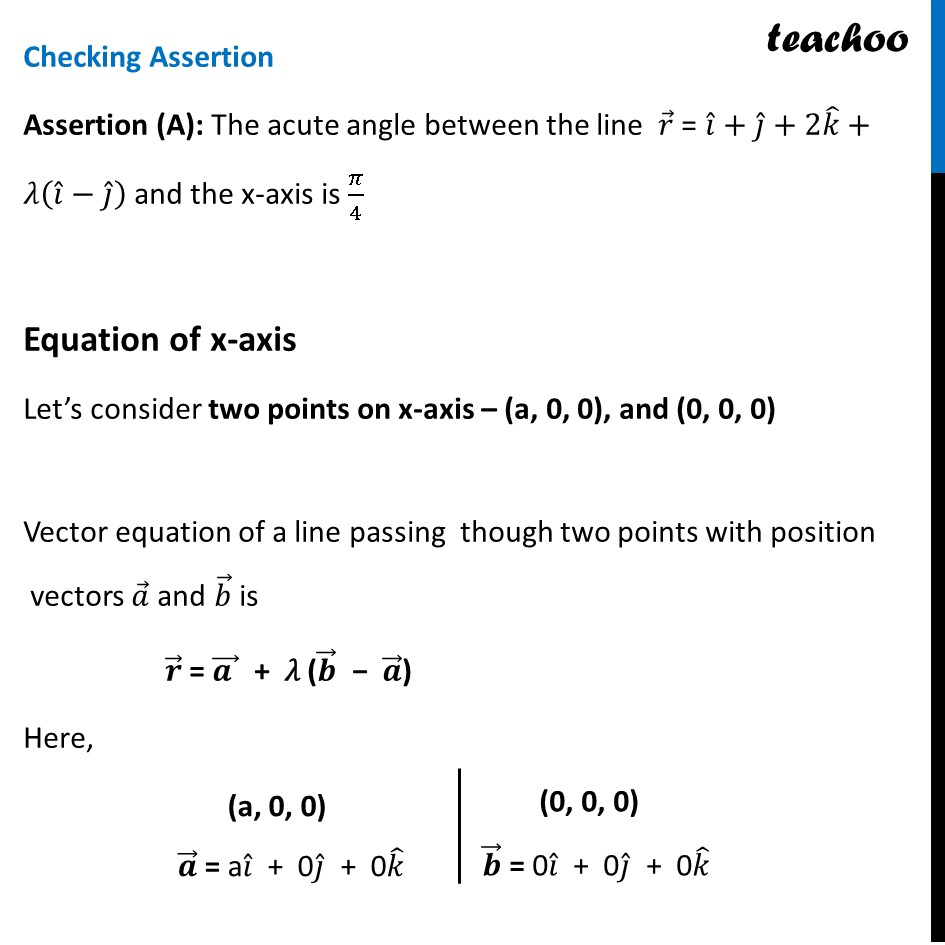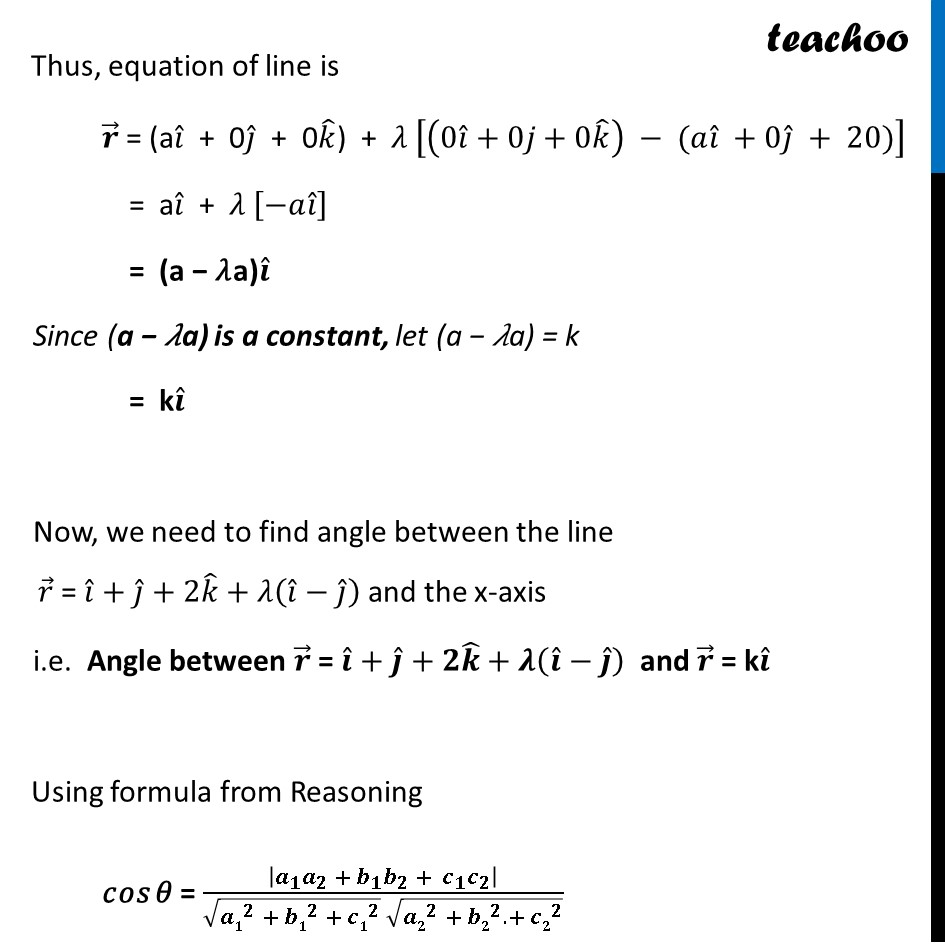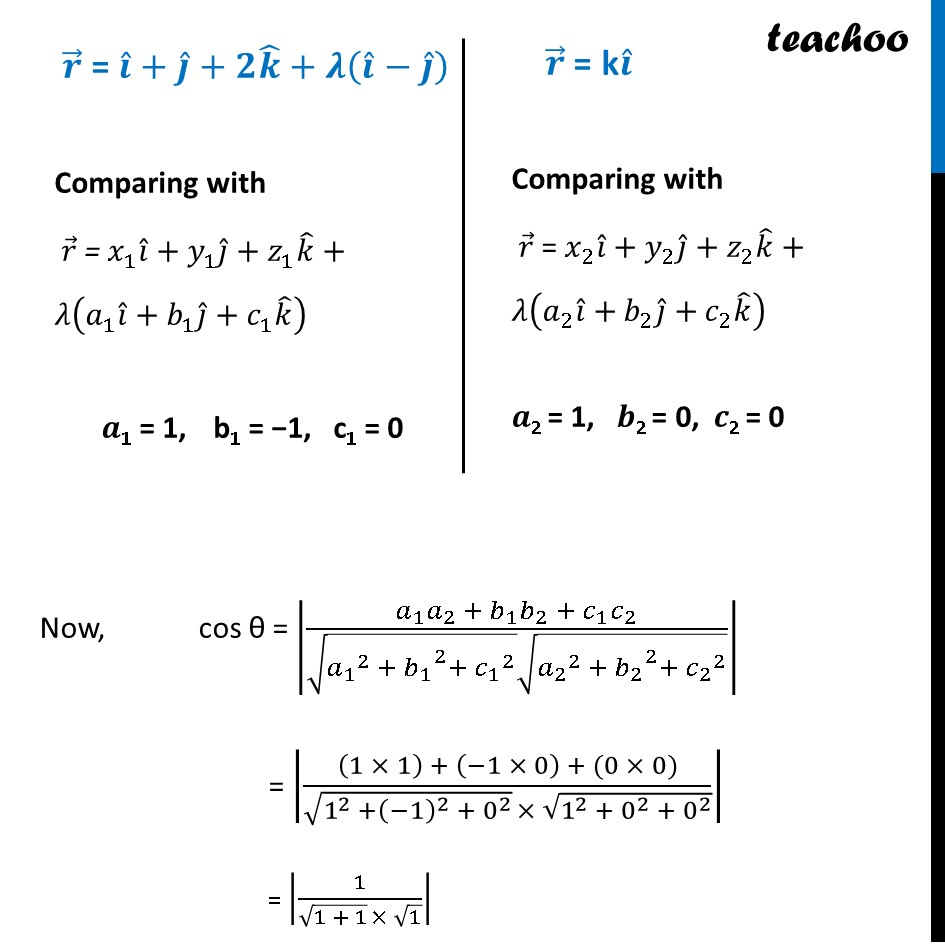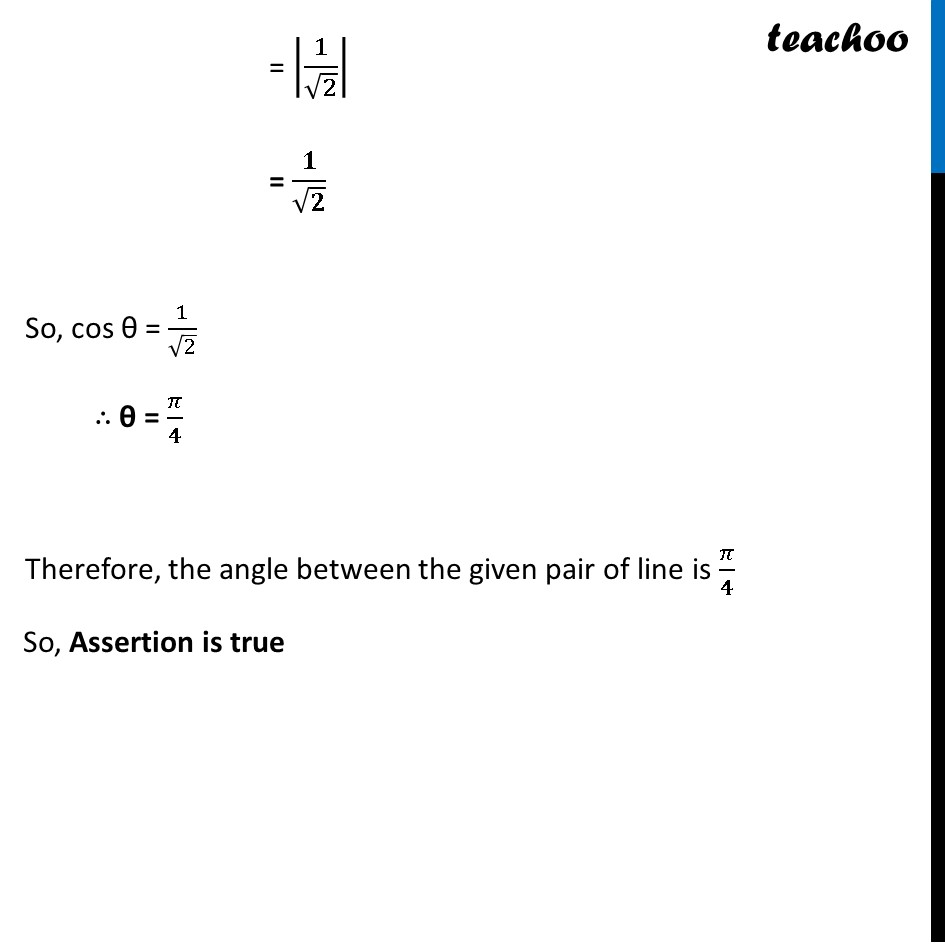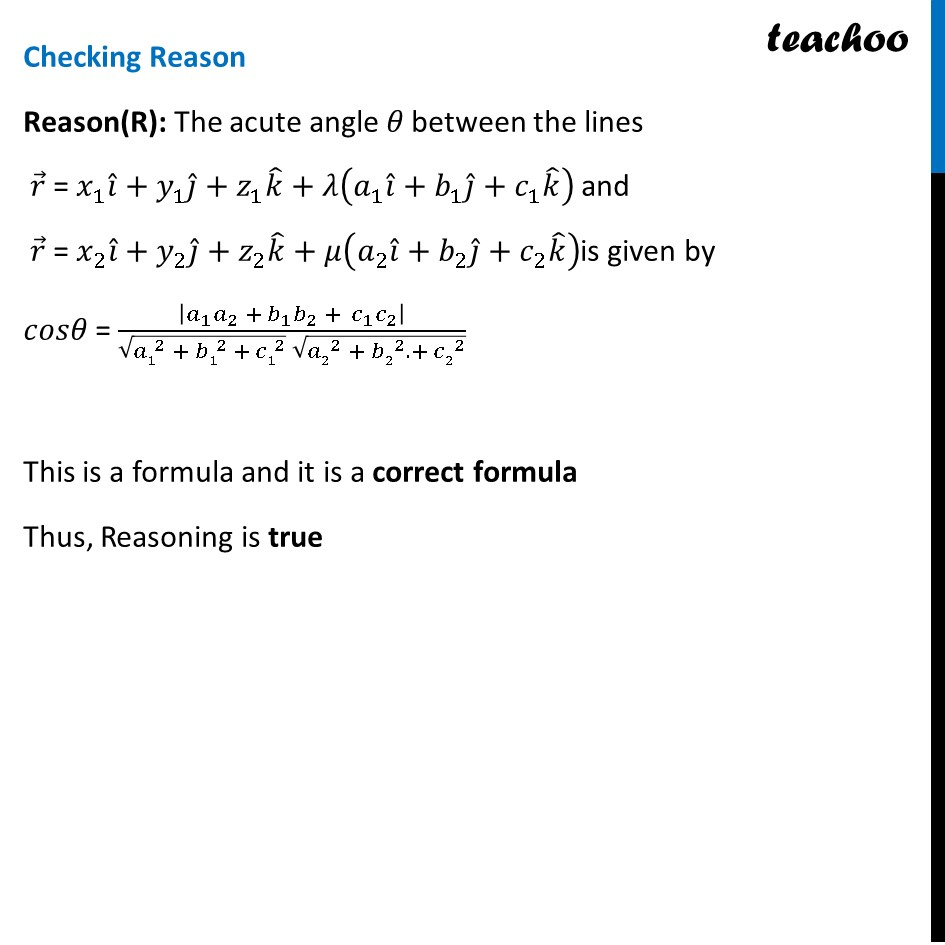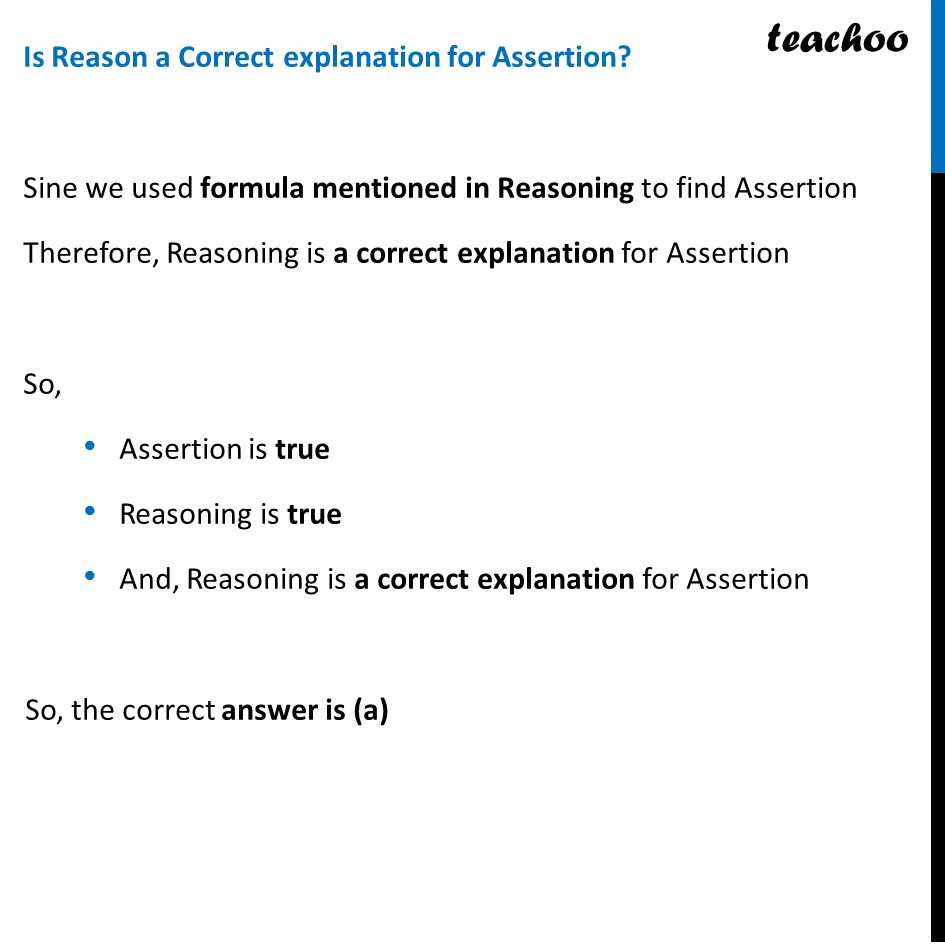Learn in your speed, with individual attention - Teachoo Maths 1-on-1 Class

### Transcript

Question 20 Assertion (A): The acute angle between the line 𝑟 ⃗ = 𝑖 ̂+𝑗 ̂+2𝑘 ̂+𝜆(𝑖 ̂−𝑗 ̂ ) and the x-axis is 𝜋/4 Reason(R): The acute angle 𝜃 between the lines 𝑟 ⃗ = 𝑥_1 𝑖 ̂+𝑦_1 𝑗 ̂+𝑧_1 𝑘 ̂+𝜆(𝑎_1 𝑖 ̂+𝑏_1 𝑗 ̂+𝑐_1 𝑘 ̂ ) and 𝑟 ⃗ = 𝑥_2 𝑖 ̂+𝑦_2 𝑗 ̂+𝑧_2 𝑘 ̂+𝜇(𝑎_2 𝑖 ̂+𝑏_2 𝑗 ̂+𝑐_2 𝑘 ̂ )is given by 𝑐𝑜𝑠𝜃 = |𝑎_1 𝑎_2 + 𝑏_1 𝑏_2 + 〖 𝑐〗_1 𝑐_2 |/(√(〖𝑎1〗^2 + 〖𝑏1〗^2 + 〖𝑐1〗^2 ) √(〖𝑎2〗^2 + 〖𝑏2〗^2. + 〖𝑐2〗^2 )) Checking Assertion Assertion (A): The acute angle between the line 𝑟 ⃗ = 𝑖 ̂+𝑗 ̂+2𝑘 ̂+𝜆(𝑖 ̂−𝑗 ̂ ) and the x-axis is 𝜋/4 Equation of x-axis Let’s consider two points on x-axis – (a, 0, 0), and (0, 0, 0) Vector equation of a line passing though two points with position vectors 𝑎 ⃗ and 𝑏 ⃗ is 𝒓 ⃗ = (𝒂 ) ⃗ + 𝜆 (𝒃 ⃗ − 𝒂 ⃗) Here, (a, 0, 0) 𝒂 ⃗ = a𝑖 ̂ + 0𝑗 ̂ + 0𝑘 ̂ (0, 0, 0) 𝒃 ⃗ = 0𝑖 ̂ + 0𝑗 ̂ + 0𝑘 ̂ Thus, equation of line is 𝒓 ⃗ = (a𝑖 ̂ + 0𝑗 ̂ + 0𝑘 ̂) + 𝜆 [(0𝑖 ̂+0𝑗+0𝑘 ̂ ) − (𝑎𝑖 ̂ +0𝑗 ̂ + 20)] = a𝑖 ̂ + 𝜆 [−𝑎𝑖 ̂ ] = (a − 𝜆a)𝒊 ̂ Since (a − 𝜆a) is a constant, let (a − 𝜆a) = k = k𝒊 ̂ Now, we need to find angle between the line 𝑟 ⃗ = 𝑖 ̂+𝑗 ̂+2𝑘 ̂+𝜆(𝑖 ̂−𝑗 ̂ ) and the x-axis i.e. Angle between 𝒓 ⃗ = 𝒊 ̂+𝒋 ̂+𝟐𝒌 ̂+𝝀(𝒊 ̂−𝒋 ̂ ) and 𝒓 ⃗ = k𝒊 ̂ Using formula from Reasoning 𝑐𝑜𝑠 𝜃 = |𝒂_𝟏 𝒂_𝟐 + 𝒃_𝟏 𝒃_𝟐 + 〖 𝒄〗_𝟏 𝒄_𝟐 |/(√(〖𝒂𝟏〗^𝟐 + 〖𝒃𝟏〗^𝟐 + 〖𝒄𝟏〗^𝟐 ) √(〖𝒂𝟐〗^𝟐 + 〖𝒃𝟐〗^𝟐. + 〖𝒄𝟐〗^𝟐 )) Thus, equation of line is 𝒓 ⃗ = (a𝑖 ̂ + 0𝑗 ̂ + 0𝑘 ̂) + 𝜆 [(0𝑖 ̂+0𝑗+0𝑘 ̂ ) − (𝑎𝑖 ̂ +0𝑗 ̂ + 20)] = a𝑖 ̂ + 𝜆 [−𝑎𝑖 ̂ ] = (a − 𝜆a)𝒊 ̂ Since (a − 𝜆a) is a constant, let (a − 𝜆a) = k = k𝒊 ̂ Now, we need to find angle between the line 𝑟 ⃗ = 𝑖 ̂+𝑗 ̂+2𝑘 ̂+𝜆(𝑖 ̂−𝑗 ̂ ) and the x-axis i.e. Angle between 𝒓 ⃗ = 𝒊 ̂+𝒋 ̂+𝟐𝒌 ̂+𝝀(𝒊 ̂−𝒋 ̂ ) and 𝒓 ⃗ = k𝒊 ̂ Using formula from Reasoning 𝑐𝑜𝑠 𝜃 = |𝒂_𝟏 𝒂_𝟐 + 𝒃_𝟏 𝒃_𝟐 + 〖 𝒄〗_𝟏 𝒄_𝟐 |/(√(〖𝒂𝟏〗^𝟐 + 〖𝒃𝟏〗^𝟐 + 〖𝒄𝟏〗^𝟐 ) √(〖𝒂𝟐〗^𝟐 + 〖𝒃𝟐〗^𝟐. + 〖𝒄𝟐〗^𝟐 )) 𝒓 ⃗ = 𝒊 ̂+𝒋 ̂+𝟐𝒌 ̂+𝝀(𝒊 ̂−𝒋 ̂ ) Comparing with 𝑟 ⃗ = 𝑥_1 𝑖 ̂+𝑦_1 𝑗 ̂+𝑧_1 𝑘 ̂+𝜆(𝑎_1 𝑖 ̂+𝑏_1 𝑗 ̂+𝑐_1 𝑘 ̂ ) 𝒂1 = 1, b1 = −1, c1 = 0 𝒓 ⃗" = k" 𝒊 ̂ Comparing with 𝑟 ⃗ = 𝑥_2 𝑖 ̂+𝑦_2 𝑗 ̂+𝑧_2 𝑘 ̂+𝜆(𝑎_2 𝑖 ̂+𝑏_2 𝑗 ̂+𝑐_2 𝑘 ̂ ) 𝒂2 = 1, 𝒃2 = 0, 𝒄2 = 0 Now, cos θ = |(𝑎_1 𝑎_2 + 𝑏_1 𝑏_2 +〖 𝑐〗_1 𝑐_2)/(√(〖𝑎_1〗^2 + 〖𝑏_1〗^2+ 〖𝑐_1〗^2 ) √(〖𝑎_2〗^2 +〖〖 𝑏〗_2〗^2+ 〖𝑐_2〗^2 ))| = |((1 × 1) + (−1 × 0) + (0 × 0))/(√(1^2 +(−1)^2 + 0^2 ) × √(1^2 + 0^2 + 0^2 ))| = |1/(√(1 + 1) × √1)| = |1/√2| = 𝟏/√𝟐 So, cos θ = 1/√2 ∴ θ = 𝜋/𝟒 Therefore, the angle between the given pair of line is 𝜋/𝟒 So, Assertion is true Checking Reason Reason(R): The acute angle 𝜃 between the lines 𝑟 ⃗ = 𝑥_1 𝑖 ̂+𝑦_1 𝑗 ̂+𝑧_1 𝑘 ̂+𝜆(𝑎_1 𝑖 ̂+𝑏_1 𝑗 ̂+𝑐_1 𝑘 ̂ ) and 𝑟 ⃗ = 𝑥_2 𝑖 ̂+𝑦_2 𝑗 ̂+𝑧_2 𝑘 ̂+𝜇(𝑎_2 𝑖 ̂+𝑏_2 𝑗 ̂+𝑐_2 𝑘 ̂ )is given by 𝑐𝑜𝑠𝜃 = |𝑎_1 𝑎_2 + 𝑏_1 𝑏_2 + 〖 𝑐〗_1 𝑐_2 |/(√(〖𝑎1〗^2 + 〖𝑏1〗^2 + 〖𝑐1〗^2 ) √(〖𝑎2〗^2 + 〖𝑏2〗^2. + 〖𝑐2〗^2 )) This is a formula and it is a correct formula Thus, Reasoning is true Is Reason a Correct explanation for Assertion? Sine we used formula mentioned in Reasoning to find Assertion Therefore, Reasoning is a correct explanation for Assertion So, Assertion is true Reasoning is true And, Reasoning is a correct explanation for Assertion So, the correct answer is (a)